Commutation and anti-commutation relationships, representation of

representation of commutation and anti-commutation relations

A linear weakly-continuous mapping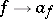,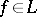, from a pre-Hilbert spaceinto a set of operators acting in some Hilbert spacesuch that either the commutation relations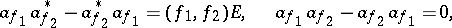(1)

or the anti-commutation relations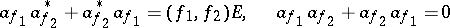(2)

hold, where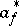,, is the adjoint of the operator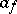in,is the identity operator inand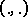is the scalar product in.

In the case whenis finite-dimensional, all irreducible representations both of relation (1) and of (2) are unitarily equivalent. In the case of an infinite-dimensional space there are infinitely many distinct (not unitarily equivalent) irreducible representations of (1) and (2); for complete separablethey are described in .

Operators,, satisfying (1) and (2) form the basis of the so-called second quantization formalism (whereis usually called the annihilation operator of a particle in stateandis the creation operator of this particle), often used in the study of quantum physical systems with a large number of degrees of freedom. However in second quantization one uses mainly the so-called Fock [Fok] representation of the commutation and anti-commutation relations; these are irreducible representations with as index spacea separable Hilbert space, while in the spacethere exists a so-called vacuum vector that is annihilated by all operators,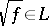.